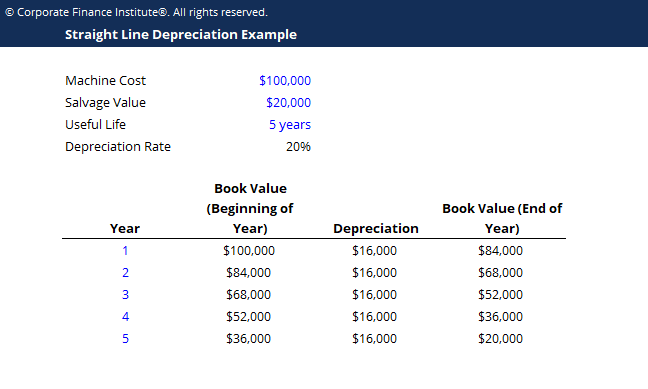# Straight Line Depreciation Template

## Straight Line Depreciation Template

This straight line depreciation template demonstrates how to calculate depreciation expense using the straight line depreciation method.

Below is a screenshot of the straight line depreciation template:### Download the Free Template

Enter your name and email in the form below and download the free template now!

### Straight Line Depreciation Template

Download the free Excel template now to advance your finance knowledge!

With the straight line depreciation method, the value of an asset is reduced uniformly over each period until it reaches its salvage value. Straight line depreciation is the most commonly used and straightforward depreciation method for allocating the cost of an asset.  It is calculated by dividing the cost by the useful life of the asset.

### Formula for Straight Line Depreciation

The formula for straight line depreciation is as follows:

Annual Depreciation Expense = (Cost of the Asset − Salvage Value) / Useful Life of the Asset

Where:

Cost of the asset is the purchase price of the asset

Salvage value is the value of the asset at the end of its useful life

Useful life of asset represents the number of periods in which the asset is expected to be used by the company

Additionally, the straight line depreciation rate can be calculated as follows:

Straight Line Depreciation Rate = Annual Depreciation Expense / (Cost of the Asset − Salvage Value)

### More Free Templates

For more resources, check out our business templates library to download numerous free Excel modeling, PowerPoint presentation and Word document templates.

• Excel Modeling Templates
• PowerPoint Presentation Templates
• Transaction Document Templates

### Financial Analyst Certification

Become a certified Financial Modeling and Valuation Analyst (FMVA)® by completing CFI’s online financial modeling classes and training program!